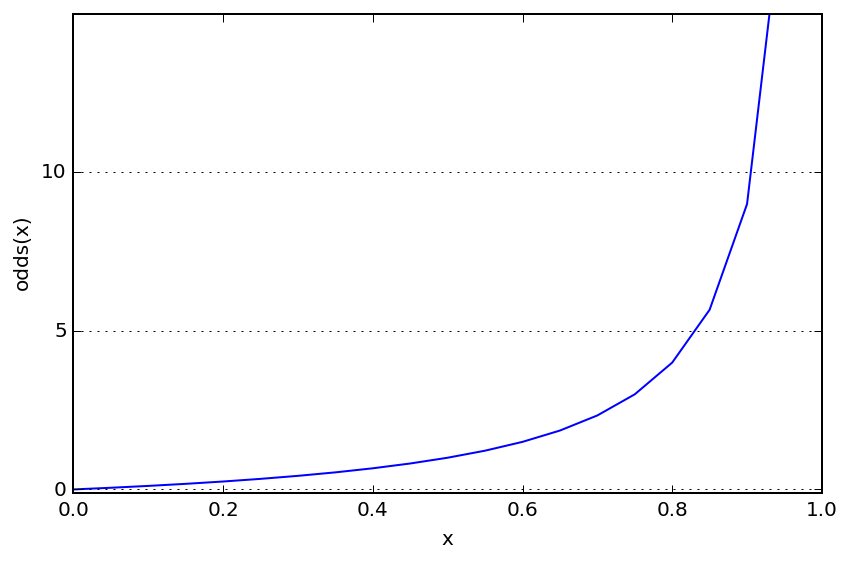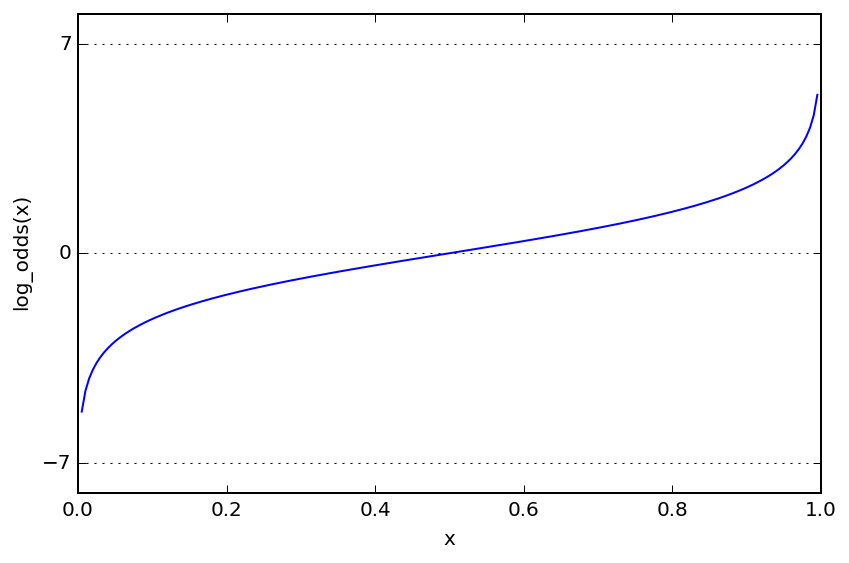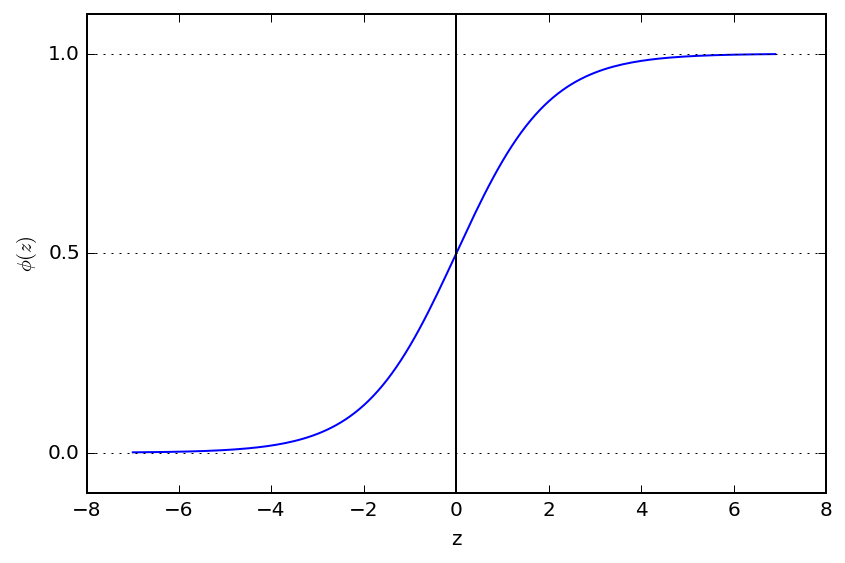# The Sigmoid Function in Logistic Regression¶

In learning about logistic regression, I was at first confused as to why a sigmoid function was used to map from the inputs to the predicted output. I mean, sure, it's a nice function that cleanly maps from any real number to a range of $-1$ to $1$, but where did it come from? This notebook hopes to explain.

## Logistic Regression¶

With classification, we have a sample with some attributes (a.k.a features), and based on those attributes, we want to know whether it belongs to a binary class or not. The probability that the output is 1 given its input could be represented as:

$$P(y=1 \mid x)$$

If the data samples have $n$ features, and we think we can represent this probability via some linear combination, we could represent this as:

$$P(y=1 \mid x) = w_o + w_1x_1 + w_2x_2 + ... + w_nx_n$$

The regression algorithm could fit these weights to the data it sees, however, it would seem hard to map an arbitrary linear combination of inputs, each would may range from $-\infty$ to $\infty$ to a probability value in the range of $0$ to $1$.

### The Odds Ratio¶

The odds ratio is a related concept to probability that can help us. It is equal to the probability of success divided by the probability of failure, and may be familiar to you if you ever look at betting lines in sports matchups:

$$odds(p) = \frac{p}{1-p}$$

Saying, "the odds of the output being 1 given an input" still seems to capture what we're after. However, if we plot the odds function from 0 to 1, there's still a problem:

In :
import matplotlib.pyplot as plt
import numpy as np
%matplotlib inline
%config InlineBackend.figure_format = 'retina'

def odds(p):
return p / (1 - p)

x = np.arange(0, 1, 0.05)
odds_x = odds(x)

plt.plot(x, odds_x)
plt.axvline(0.0, color='k')
plt.ylim(-0.1, 15)
plt.xlabel('x')
plt.ylabel('odds(x)')

# y axis ticks and gridline
plt.yticks([0.0, 5, 10])
ax = plt.gca()
ax.yaxis.grid(True)

plt.tight_layout()
plt.show()### Log Odds¶

An arbitrary linear combination of the input features may still be less than zero. However, if we take the log of the odds ratio, we now get something that ranges from $-\infty$ to $\infty$

In :
def log_odds(p):
return np.log(p / (1 - p))

x = np.arange(0.005, 1, 0.005)
log_odds_x = log_odds(x)

plt.plot(x, log_odds_x)
plt.axvline(0.0, color='k')
plt.ylim(-8, 8)
plt.xlabel('x')
plt.ylabel('log_odds(x)')

# y axis ticks and gridline
plt.yticks([-7, 0, 7])
ax = plt.gca()
ax.yaxis.grid(True)

plt.tight_layout()
plt.show()Having a linear combination of arbitary features map to the log_odds function allows for any possible input values for each $x_i$ and still represents conceptually what we are trying to represent: that a linear combination of inputs is related to the liklihood that a sample belongs to a certain class.

Note: the log of the odds function is often called "the logistic" function.

So now we have:

$$\text{log_odds}(P(y=1 \mid x)) = w_o + w_1x_1 + w_2x_2 + ... + w_nx_n$$

If we still want to get plain old $P(y=1 \mid x)$ we can by taking the inverse of the log_odds function.

## The Sigmoid¶

Let's find the inverse of the log_odds function:

Starting with:

$y = log(\frac{x}{1-x})$

and swapping $y$ and $x$ and solving for $y$

$x = log(\frac{y}{1-y})$
$e^x = \frac{y}{1-y}$
$y = (1-y)*e^x$
$y = e^x - y*e^x$
$y + ye^x = e^x$
$y*(1 + e^x) = e^x$
$y = \frac{e^x}{1+e^x}$
$y = \frac{1}{\frac{1}{e^x} + 1}$
$y = \frac{1}{1 + e^{-x}}$

Let's use $\phi$ to represent this function and plot it to get a sense of what it looks like:

In :
def inverse_log_odds(z):
return 1.0 / (1.0 + np.exp(-z))

z = np.arange(-7, 7, 0.1)
phi_z = inverse_log_odds(z)

plt.plot(z, phi_z)
plt.axvline(0.0, color='k')
plt.ylim(-0.1, 1.1)
plt.xlabel('z')
plt.ylabel('$\phi (z)$')

# y axis ticks and gridline
plt.yticks([0.0, 0.5, 1.0])
ax = plt.gca()
ax.yaxis.grid(True)

plt.tight_layout()
plt.show()The inverse form of the logistic function is looks kind of like an S, which, I've read, is why it's called a Sigmoid function.

So going back to our:

$$\text{log_odds}(P(y=1 \mid x)) = w_o + w_1x_1 + w_2x_2 + ... + w_nx_n$$

If we call $w_o + w_1x_1 + w_2x_2 + ... + w_nx_n = w^Tx$ simply $z(x)$:

$$\text{log_odds}(P(y=1 \mid x)) = z(x)$$

And taking the inverse:

$$P(y=1 \mid x) = \phi(z) = \dfrac{1}{1 + e^{-z}}$$

and there you have it: Logistic Regression fits weights so that a linear combination of its inputs maps to the log odds the output being equal to 1.